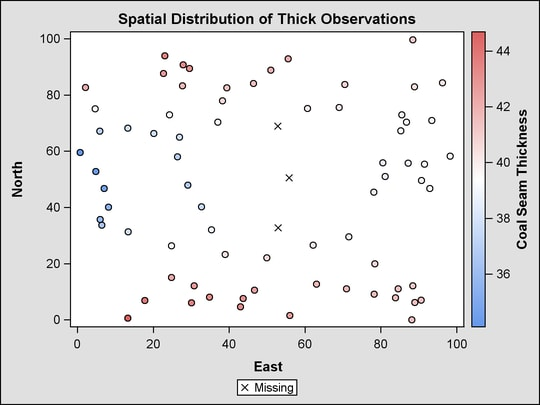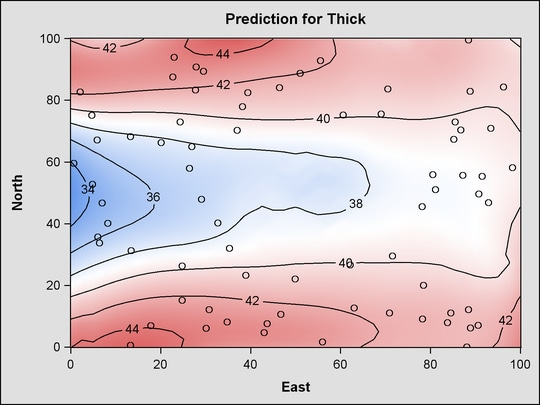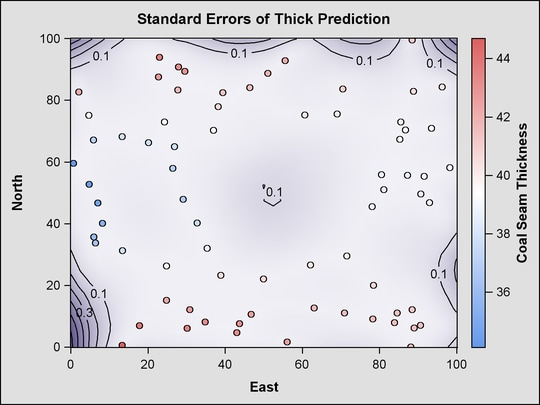Statistical Graphics Using ODS
 Contour Plots with PROC KRIGE2D

This example is taken from Example 46.2 of Chapter 46, The KRIGE2D Procedure. The following statements create a SAS data set that contains measurements of coal seam thickness:

```data thick;
input East North Thick @@;
label Thick='Coal Seam Thickness';
datalines;
0.7  59.6  34.1   2.1  82.7  42.2   4.7  75.1  39.5

... more lines ...

;
```

The following statements set the output style to DEFAULT and run PROC KRIGE2D:

```ods listing style=default;
ods graphics on;

proc krige2d data=thick outest=predictions
plots=(observ(showmissing)
coordinates xc=East yc=North;
predict var=Thick r=60;
model scale=7.2881 range=30.6239 form=gauss;
grid x=0 to 100 by 2.5 y=0 to 100 by 2.5;
run;
```

The PLOTS=OBSERV(SHOWMISSING) option produces a scatter plot of the data along with the locations of any missing data. The PLOTS=PRED option produces maps of the kriging predictions and standard errors. Two instances of the PLOTS=PRED option are specified with suboptions that customize the plots. The results are shown in Figure 21.7.

Figure 21.7 PROC KRIGE2D Results Using the DEFAULT StylePrevious Page | Next Page | Top of Page